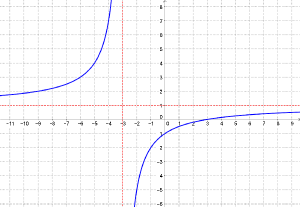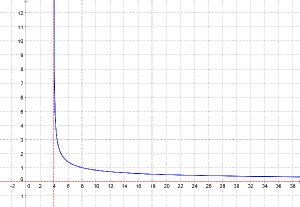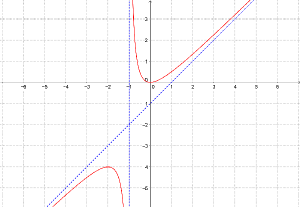# Finding the Range of a Function

Recall that the domain of a function is the set of all valid input values (x), and the range is the set of all possible output values (y). It is reasonably easy to find the domain: look for what could make it impossible to evaluate, such as dividing by zero or taking the square root of a negative number, and exclude those. But finding the range is an entirely different problem. There is no one technique that will work for all functions. But there are several methods that work in reasonably nice cases.

## A rational function

We have collected some of our answers on both domain and range in a “selected answers” page here; I will look at several answers specifically dealing with range, and refer to more in passing.

First, here is one from 2002:

Range

If f(x) = (x-3)/(x+3), I know the domain to be all Reals except for -3. The range, however, is all Reals except for 1. I don't know how they get the 1 algebraically.

Teresa understands the domain: $$x$$ can be any real number that does not make the denominator, $$\left(x+3\right)$$, equal to zero, and therefore anything except $$-3$$. But how to find the range? Since she knew the answer, I could use her problem as an example, demonstrating the whole method. The key idea is to use the fact that the domain of a function is the same as the range of its inverse. (This is because the inverse must take any possible output from the function and turn it into the corresponding input; so the set of outputs of the original function is the same as the set of inputs of the inverse.) This is true even when the inverse is not actually a function, just a relation (which may have more than one value), although not all textbooks talk about this.

You can also do all this without ever thinking about inverses! All we are doing, as you see below, is solving to find $$x$$ when you know $$y$$, and then looking at the result to see for what values of y this can be done.

Hi, Teresa.

The usual way to do this is to try to invert the function; then the domain of the inverse is the range of the function. That is, we solve for x:

y = (x-3)/(x+3)
y(x+3) = x-3
xy + 3y = x - 3
xy - x = -3 - 3y
x(y - 1) = -(3 + 3y)
x = (3 + 3y)/(1 - y)

Now we can see immediately that y can be anything but 1. Since for any y except 1, there is an x, we know that the original function can take any value except 1.

Having done that, we can look back at the original function and see why it can't be 1. If we had

(x-3)/(x+3) = 1

then x-3 = x+3, which is impossible.

I showed all the steps of solving, because solving a rational function like this often stymies students when they first confront it. The key steps are to collect all the x‘s on one side, and then factor out the $$x$$ so you can solve for it.

You may also notice that although I talked about finding an inverse, I did not swap $$x$$ and $$y$$ as is often taught. I discussed this recently; for this application, I find it useful to keep $$x$$ and $$y$$ having the same meaning throughout, to avoid confusion.

Let’s make a graph of the function, and verify what the domain and range are:Yes, the domain excludes $$-3$$, and the range excludes $$1$$.

Evidently Teresa understood that well; but two days later, she wrote back with a more interesting function:

In f(x) = 2/sqrt(x-4), how do you find the range?

Since I had shown the general method, I wanted Teresa to at least make a start, using what I had taught; but I acknowledged that she would discover some new challenges:

Did you try using the method I showed you last time? We can help a lot better if you show us your work so we can see why you are having trouble - just as a medical doctor wants to see where it hurts, a math doctor wants to see what doesn't work.

Solve

y = 2/sqrt(x-4)

for x, and see what values of y do not allow you to find an x. Those values are excluded from the range.

In this case there is an extra issue: the square root is always taken as the POSITIVE root, which limits y further.

She responded as well as I hoped she would, starting out well:

Thanks for being patient with me.  I worked f(x)= 2/sqrt(x-4) like this:

y(sqrt(x-4)= 2.

Then, I moved the y:

sqrt(x-4)= 2/y.

Then I squared both sides and obtained

x-4 = 4/y^2

This is where my question arises.  If I then move the four on the left side of the equation and then solve for one y, I get an imaginary number.  Was that what you were referring to that would always be positive?  That would give a 4 + 4, so I am lost at that point.

She got a little lost here at the end, losing sight of the goal. The next step really is pretty easy, but then the complications set in, so I chose to take it all the rest of the way, in steps. First, the easy part:

You've done well so far. Remember you are solving for x, and you get

x = 4 + 4/y^2

You don't want to solve for y now; the purpose of solving for x was to see for which values of y you can find an x. Look at this equation, and you see that you can put any value of y except for 0 into it; the x you get out is just the x you could put into the original equation to get that value of y. So f(x) can take any value but 0.

The domain of this inverse function is all real numbers except zero, so we seem to be done …

But ...

Let's try an example or two. If y = 1, we get x = 4 + 4/1 = 8, and

f(8) = 2/sqrt(8-4) = 2/2 = 1

so we did in fact show that 1 is in the range. Now let y = -1. We find that

x = 4 + 4/(-1)^2 = 4 + 4/1 = 8

But that's the same x we got for y = 1, and we know that f(8) is not -1. So what happened?

If $$y = -1$$, then $$x = 8$$; but $$f(8) = 1$$, not $$-1$$. There was something I deliberately ignored in my initial work (and likely would have accidentally ignored if I had not been thinking carefully from the start – I had mentioned it when I first told Teresa what to do):

When you square y, both 1 and -1 give the same result. Another way to say that is that 1 and -1 are both square roots of 1. But in the original equation, we had the square root _function_, which is defined as the POSITIVE square root only. So

f(x) = 2/sqrt(x-4)

always gives the positive root, and so f(x) has to be always positive. That's why we don't get -1 out of it when we put 8 in, even though both 2 and -2 are square roots of 4.

So the answer is that the range of this function is all positive real numbers: zero is excluded because we couldn't divide by y^2 in our inverse, and negative numbers are excluded by the square root function.

In practice, when I am thinking carefully, I try to write explicitly the condition that is implied by the radical, and come back to that at the end of my work: $$\displaystyle y = \frac{2}{\sqrt{x-4}}, y \ge 0$$

Now it is clear that $$y$$ is not any real number except zero, but any non-negative number except zero – that is, any positive number.

Let’s look at the graph of this function:The domain and range are as we expected. (You did see that the domain was all real numbers greater than 4, right?)

For another function  similar to this, see

Domain and Range the Inverted Way

## A harder rational function

Here is one that is a little trickier:

Rational Function Range-Finding, with and without Calculus

Finding the range of a rational function is not always easy or intuitive -- particularly when the degree of the polynomial in the numerator is greater than that in the denominator.

An example would be

f(x) = (x^2)/(x + 1)

How can I find the range of this function without using a graphing calculator?

When we draw a graph of the function, a gap appears between two symmetrical curves. How can I find where that curve starts or ends algebraically, i.e., without a graphing calculator?

If I knew that, then I could find the range relative to the y-axis.

In the example above, the vertical asymptote is x = -1. Because the degree of the polynomial in the numerator is greater than that in the denominator by one, the "horizontal" asymptote is a line. By long division, we find that the line is y = x - 1. Now the domain is all R except -1.

But the range? No clue.

I've worked every part of the function except the range. I just don't know how to find it. I've searched everywhere for an algebraic method to find the range of this special case of rational functions, but I haven't found any. Even my teacher in school, who has a PhD in math, couldn't give me an answer.

Here is the graph, so we can see what Ibrahim is talking about:Without trusting the numbers we see on the graph, how can we know the boundaries of the range?

My first observation is that this is not really too hard for a teacher; but it may well be too hard to explain to a student!

Hi, Ibrahim.

I suspect your teacher has no answer in one of two senses: either that you are not yet fully prepared for an analytic approach to this particular problem; or, more likely, that no general method will work for any rational function of this sort.

Having said all that, this particular example is actually rather easy. I can think of several ways to go about it, and they all work for this one, but wouldn't work for a harder case (higher degree).

## Using calculus

One method is to use calculus to determine specifics about the graph (or, without any graphing tool, to use calculus alone to sketch the graph, with all important points known). Even this wouldn’t work for all functions, but it works well here.

The first thing I think of is calculus. You can find out, without too much trouble, where the graph is horizontal, which gives the location and values of the local minimum (x = 0, y = 0) and the local maximum (x = -2, y = -4). Then you can see the range that your graphing calculator showed you -- namely,

(-inf, -4] U [0, inf)

I didn’t go into this in detail, assuming he has not learned calculus. But let’s quickly look a little deeper, in case you have. We have $$\displaystyle y = \frac{x^2}{x+1}$$; I would simplify this by long division to get $$\displaystyle y = x – 1 + \frac{1}{x+1}$$, and then differentiate to get $$\displaystyle y’ = 1 – \frac{1}{(x+1)^2}$$. This is zero when $$(x+1)^2 = 1$$, namely at $$x = 0$$ or $$-2$$; and the function is undefined at $$x = -1$$. The derivative turns out to be negative between $$-2$$ and $$0$$, and positive elsewhere, which leads us to conclude that there is a local maximum at $$\left(-2, -4 \right)$$, and a local minimum at $$\left(0, 0 \right)$$. All of this is what you would do to graph the function; but even without graphing, it tells us that the domain is, as stated above, $$\left(-\infty, -4\right] \cup \left[0, \infty\right)$$.

## Inverting again

Assuming you don't know the little bit of calculus that is needed to do that, my next thought is to try inverting the function, as the range of a function is the domain of its inverse. Of course, the inverse here will not be a function. But if it is possible to solve for x (giving multiple solutions), you can then find for what values of y there is a solution. Those values of y are the range of our function.

So, again, we want to try solving for $$x$$, and determine for what values of $$y$$ this is possible. I just gave general instructions for this:

That can be done in this case. Solve for x by multiplying both sides by (x + 1), which will give -- treating y as a constant -- a quadratic equation in x. Apply the quadratic formula, and you have two values of x for any y for which the discriminant is not negative. So your range will be the set of y for which that discriminant is greater than or equal to zero.

Let’s carry out the details:

$$\displaystyle y = \frac{x^2}{x+1}$$

$$y\left(x+1\right) = x^2$$

$$yx + y = x^2$$

$$x^2- yx – y = 0$$

$$\displaystyle \frac{y \pm \sqrt{y^2 + 4y}}{2}$$

The discriminant, $$y^2 + 4y$$, is non-negative in the interval we found previously, $$\left(-\infty, -4\right] \cup \left[0, \infty\right)$$. This is the range: the set of values of $$y$$ for which it is possible to find a value of $$x$$ that yields that $$y$$.

This was a little harder than the first rational function we worked with, but quite reasonable.

## Guess and prove

I added one more idea: That without calculus or other elaborate tools, we can roughly sketch the graph, guess the range, and then prove that algebraically. Sometimes this is the best we can do:

One more thing you could do is to sketch a graph by hand and guess that (0, 0) is the local minimum on the right branch of the graph. Then you can show that this is true by considering the sign of y for small positive x, and for small negative x:

When      x > 0, x^2/(x + 1)  is positive
When -1 < x < 0, x^2/(x + 1)  is positive

Since when x = 0, x^2/(x + 1) = 0, that's the minimum! Then you can use symmetry (or another guess) to find the local maximum.

This work with signs is essentially what we do in solving rational inequalities, as we have done earlier.

For a couple other examples of finding a range, see the following pages. In the first, Doctor Anthony uses ad-hoc reasoning somewhat like my last suggestion; in the second, Doctor Fenton finds both domain and range for a simple polynomial function, ending up by solving for $$x$$ as we have been doing:

How to Find the Range of a Function

Finding The Domain and Range of a Function

## Why domain and range?

Here is a final bonus: A student asked why we call them domain and range, and I made some interesting observations about the words:

Domain and Range of Functions

I am learning in school about the domain and range of functions. Why are they called domain and range? And where did they come from?

"Domain" and "range" are just two different words for "how far something extends"; specifically, a king's domain is the territory he controls, and an animal's range is the region it wanders through. So it makes some sense that the set of numbers a function "controls" would be called its domain, and the set through which its value can wander is called its range.

To be more precise, Merriam-Webster, at m-w.com, defines the words (in the most relevant usage) this way:

Domain 2 : a territory over which dominion is exercised
5 : the set of elements to which a mathematical
or logical variable is limited; specifically :
the set on which a function is defined

(The word comes from the Latin word for "lordship".)

Range  3 a : a place that may be ranged over
b : an open region over which animals
(as livestock) may roam and feed
c : the region throughout which a
kind of organism or ecological
community naturally lives or occurs
8 a : the set of values a function may take on
b : the class of admissible values of a variable

(Several other uses are similar, such as the range of a weapon or a voice; the original meaning comes from the idea of animals ranging about.)

### 2 thoughts on “Finding the Range of a Function”

This site uses Akismet to reduce spam. Learn how your comment data is processed.